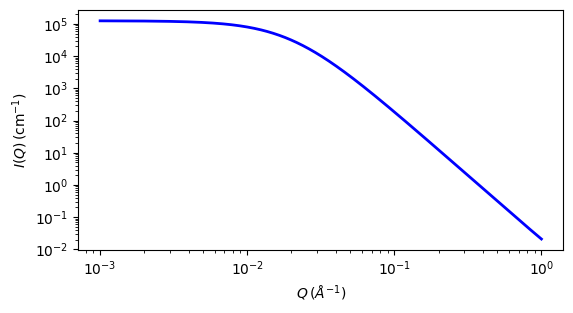# dab

DAB (Debye Anderson Brumberger) Model

Parameter

Description

Units

Default value

scale

Scale factor or Volume fraction

None

1

background

Source background

cm-1

0.001

cor_length

correlation length

50

The returned value is scaled to units of cm-1 sr-1, absolute scale.

Calculates the scattering from a randomly distributed, two-phase system based on the Debye-Anderson-Brumberger (DAB) model for such systems. The two-phase system is characterized by a single length scale, the correlation length, which is a measure of the average spacing between regions of phase 1 and phase 2. The model also assumes smooth interfaces between the phases and hence exhibits Porod behavior $$(I \sim q^{-4})$$ at large $$q$$, $$(qL \gg 1)$$.

The DAB model is ostensibly a development of the earlier Debye-Bueche model.

Definition

$I(q) = \text{scale}\cdot\frac{L^3}{(1 + (q\cdot L)^2)^2} + \text{background}$

where scale is

$\text{scale} = 8 \pi \phi (1-\phi) \Delta\rho^2$

and the parameter $$L$$ is the correlation length.

For 2D data the scattering intensity is calculated in the same way as 1D, where the $$q$$ vector is defined as

$q = \sqrt{q_x^2 + q_y^2}$Fig. 100 1D plot corresponding to the default parameters of the model.

Source

dab.py

References

1. P Debye, H R Anderson, H Brumberger, Scattering by an Inhomogeneous Solid. II. The Correlation Function and its Application, J. Appl. Phys., 28(6) (1957) 679

2. P Debye, A M Bueche, Scattering by an Inhomogeneous Solid, J. Appl. Phys., 20 (1949) 518

Source

dab.py

Authorship and Verification

• Author: